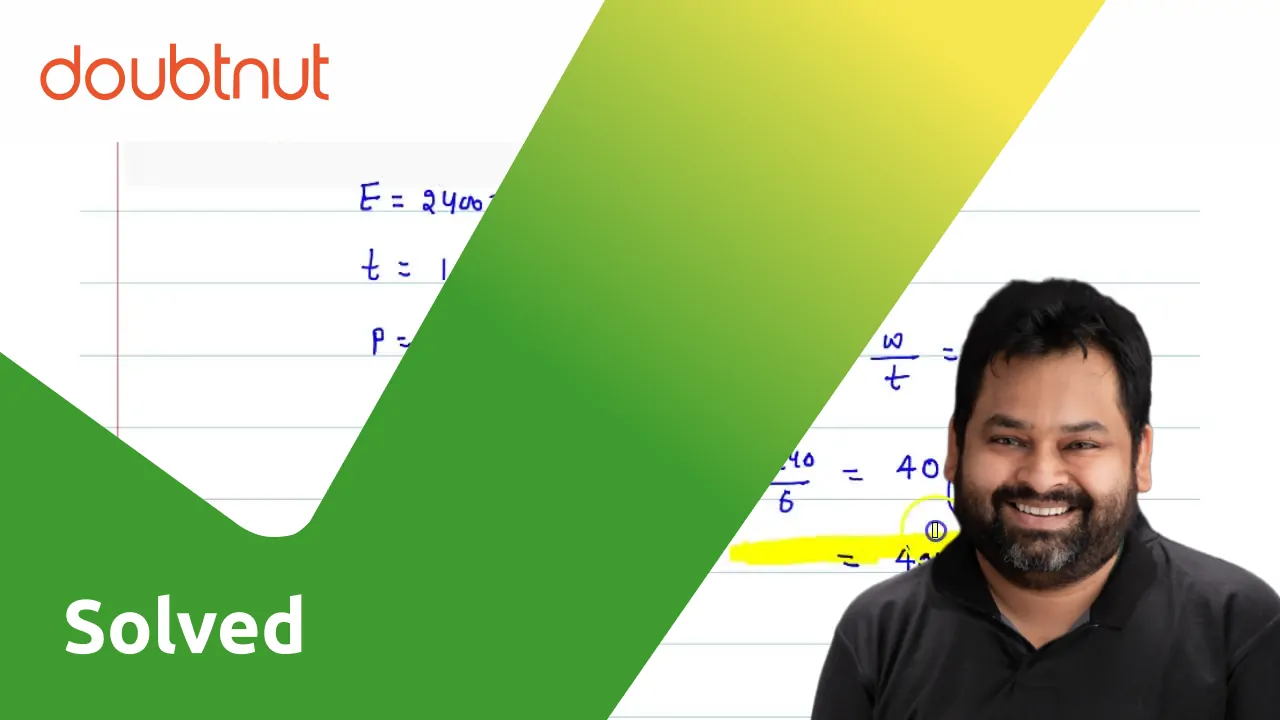Doubtnut App - Get 50 Lakh+ video solutions better than GoogleInstall Now

# Calculate the power of an electric bulb which consumers 2400 J is a minute

Text Solution
Verified by Experts

## Given Energy consumed (E)=2400J Time (t)=1 minute =60s Power (P)=? P=Wt=Et=2400J60s =40Js−1 :P=40Js−1=40W.